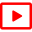## Week 15Have: evolution law. Want: invariant predictions

• 29. Discrete symmetry factorization / 26 April 2022 /
• 3 disk pinball symmetries suffice to illustrate all that is needed to factorized spectral determinants for any system with a discrete symmetry: character. Discrete symmetry tiles the state space, and dynamics can be reduced to dynamics on the fundamental domain, together with a finite matrix that keeps track of the tile the full state space trajectory lands on. We need some group theory (one needs to underatand the projection to irreducible representations) and illustrate how different classes of periodic orbits contribute to different invariant subspaces for the 3-disk pinball. Read sects. 25.2 - 25.6.Chapter 25 Discrete symmetry factorizationFactorized spectral determinantIrrep decomposition of the traceIrrep decomposition of the resolventIrrep factorization of the determinantIn 100d the most important is 99d invariant torusFactorization of D3 cycle weightsHard work builds character

• 30. Continuous symmetry factorization / 28 April 2022 bonus lecture /
• Trace formulas relate short time dynamics (unstable periodic orbits) to long time invariant state space densities (natural measure). A trace formula for a partially hyperbolic (N+ 1)-dimensional compact manifold invariant under a global continuous symmetry is derived. In this extension of “periodic orbit” theory there are no or very few periodic orbits - the relative periodic orbits that the trace formula has support on are almost never eventually periodic.Chapter 26 Continuous symmetry factorization This chapter needs a major revision, the videos are more up-to-date than the chapter itselfGroup averagingThe Great Orthonormality TheoremAverage over space and timeDiscrete time averagingContinuous time averagingIf discrete symmetry, average over itIf continuous symmetry, average over it
• Homework 15
•discrete factorization  Due 28 April 2015 (note!)Discussion forum for week 15

• Optional
•John F Gibson solves the Navier-Stokes